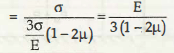K = Bulk Modulus

K is a bulk Modulus and it can be defined as the ratio between hydrostatic pressure to the Volumetric strain. And thus it can be represented as

K = Hydrostatic pressure / Volumetric strain

= σ / ϵv = σx + ϵy +ϵzE = 3k (1-2µ)

Here, σ taken as hydrostatic pressure

And ϵv = Volumetric Strain

µ = Poisson’s ratio

G = modulus of rigidity

E = modulus of elasticity.

All the above factors must be known to understand the relation in a proper way. The relationship is important to understand the various mechanical concepts related to metallic material.

Links of Next Mechanical Engineering Topics:-### Customer Reviews

My Homework Help
Rated 5.0 out of 5 based on 510 customer reviews at